# Advanced NET Programming I 6 th Lecture http

• Slides: 9
Download presentation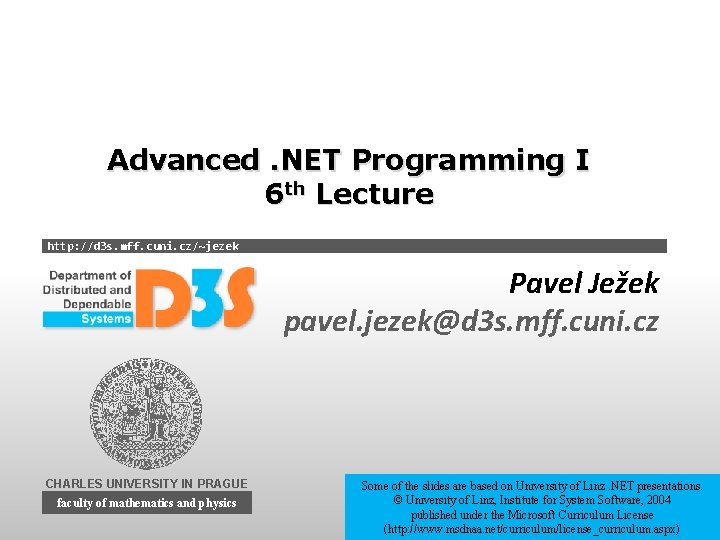Advanced. NET Programming I 6 th Lecture http: //d 3 s. mff. cuni. cz/~jezek Pavel Ježek pavel. [email protected] 3 s. mff. cuni. cz CHARLES UNIVERSITY IN PRAGUE faculty of mathematics and physics Some of the slides are based on University of Linz. NET presentations. © University of Linz, Institute for System Software, 2004 published under the Microsoft Curriculum License (http: //www. msdnaa. net/curriculum/license_curriculum. aspx)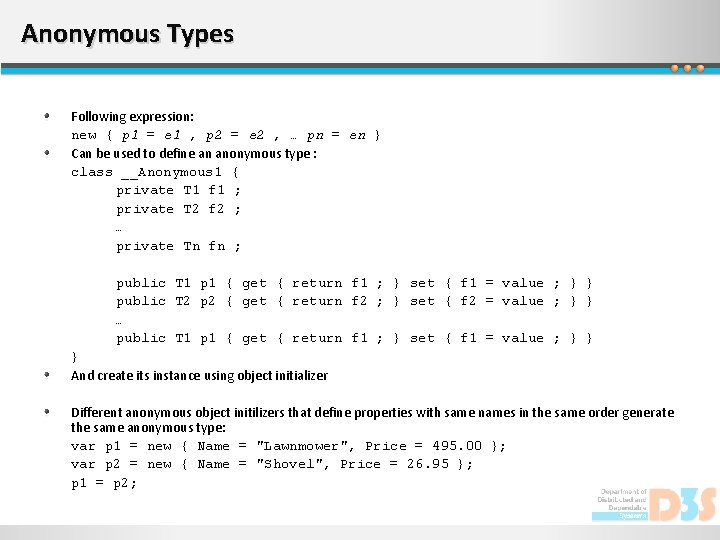Anonymous Types Following expression: new { p 1 = e 1 , p 2 = e 2 , … pn = en } Can be used to define an anonymous type : class __Anonymous 1 { private T 1 f 1 ; private T 2 f 2 ; … private Tn fn ; public T 1 p 1 { get { return f 1 ; } set { f 1 = value ; } } public T 2 p 2 { get { return f 2 ; } set { f 2 = value ; } } … public T 1 p 1 { get { return f 1 ; } set { f 1 = value ; } } } And create its instance using object initializer Different anonymous object initilizers that define properties with same names in the same order generate the same anonymous type: var p 1 = new { Name = "Lawnmower", Price = 495. 00 }; var p 2 = new { Name = "Shovel", Price = 26. 95 }; p 1 = p 2;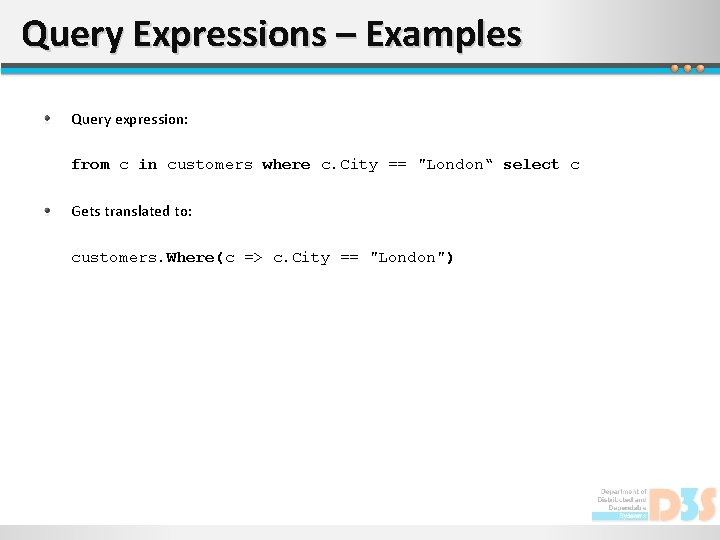Query Expressions – Examples Query expression: from c in customers where c. City == "London“ select c Gets translated to: customers. Where(c => c. City == "London")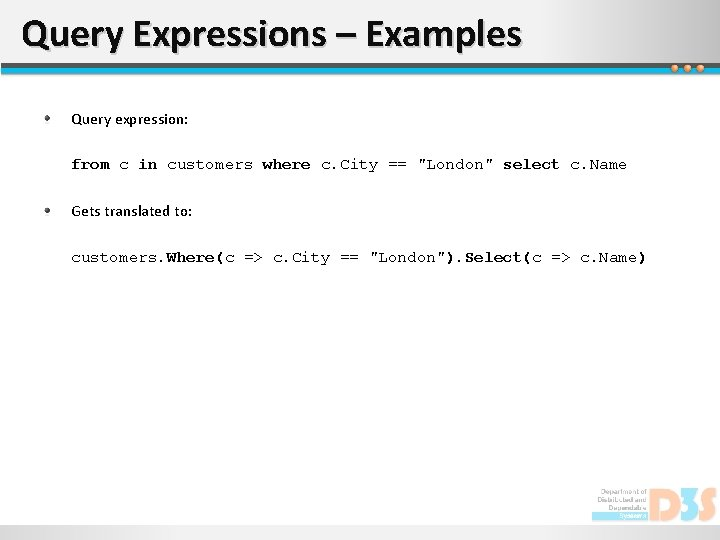Query Expressions – Examples Query expression: from c in customers where c. City == "London" select c. Name Gets translated to: customers. Where(c => c. City == "London"). Select(c => c. Name)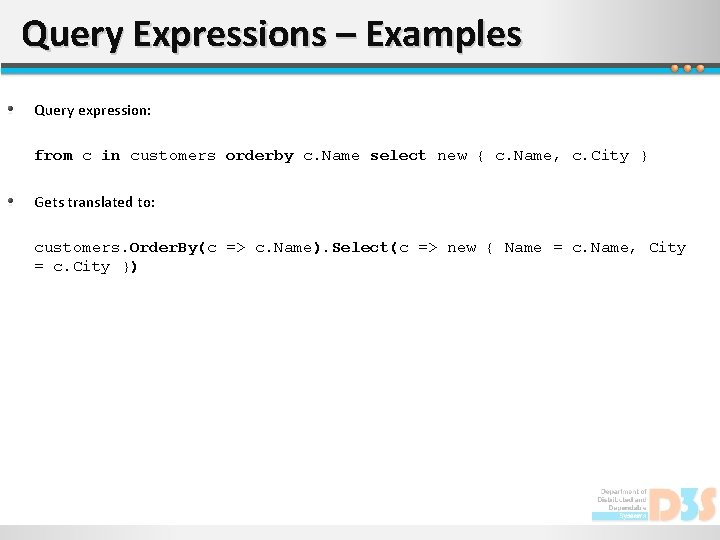Query Expressions – Examples Query expression: from c in customers orderby c. Name select new { c. Name, c. City } Gets translated to: customers. Order. By(c => c. Name). Select(c => new { Name = c. Name, City = c. City })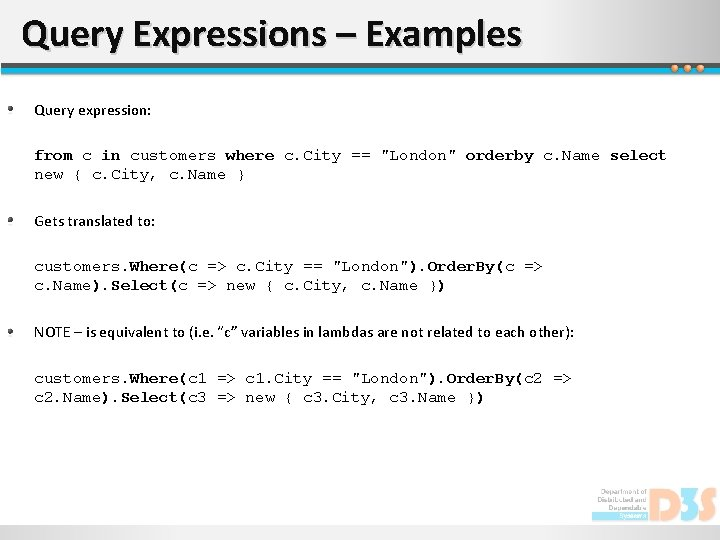Query Expressions – Examples Query expression: from c in customers where c. City == "London" orderby c. Name select new { c. City, c. Name } Gets translated to: customers. Where(c => c. City == "London"). Order. By(c => c. Name). Select(c => new { c. City, c. Name }) NOTE – is equivalent to (i. e. “c” variables in lambdas are not related to each other): customers. Where(c 1 => c 1. City == "London"). Order. By(c 2 => c 2. Name). Select(c 3 => new { c 3. City, c 3. Name })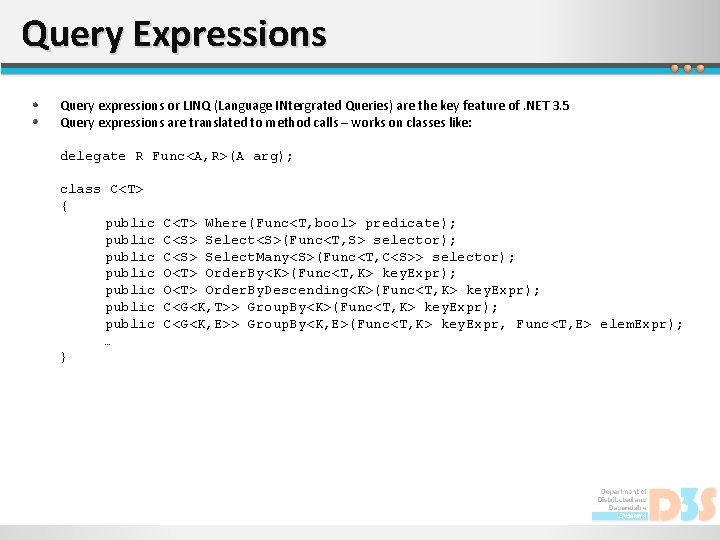Query Expressions Query expressions or LINQ (Language INtergrated Queries) are the key feature of. NET 3. 5 Query expressions are translated to method calls – works on classes like: delegate R Func<A, R>(A arg); class C<T> { public public … } C<T> Where(Func<T, bool> predicate); C<S> Select<S>(Func<T, S> selector); C<S> Select. Many<S>(Func<T, C<S>> selector); O<T> Order. By<K>(Func<T, K> key. Expr); O<T> Order. By. Descending<K>(Func<T, K> key. Expr); C<G<K, T>> Group. By<K>(Func<T, K> key. Expr); C<G<K, E>> Group. By<K, E>(Func<T, K> key. Expr, Func<T, E> elem. Expr);LINQ to Objects Set of generic extension methods (Select, Where, Order. By, etc. ) implemented for IEnumerable<T> interface (provided by static class Enumerable), example: int[] numbers = { 5, 4, 1, 3, 9, 8, 6, 7, 2, 0 }; var number. Groups = from n in numbers group n by n % 5 into g select new { Remainder = g. Key, Numbers = g }; foreach (var g in number. Groups) { Console. Write. Line( "Numbers with a remainder of {0} when divided by 5: ", g. Remainder ); Numbers with a remainder of 0 when divided by 5: foreach (int n in g. Numbers) { 5 Console. Write. Line(n); 0 Numbers with a remainder of 4 when divided by 5: } 4 9 } LINQ to * - Classes for * data access using query expressions NOTE: For any LINQ implementation it is commonly expected (but not enforced [see Linq. To. Nothing example]), that the “resulting” type of any query implements at least the IEnumerable interface. Numbers with a remainder of 1 when divided by 5: 1 6 Numbers with a remainder of 3 when divided by 5: 3 8 Numbers with a remainder of 2 when divided by 5: 7 2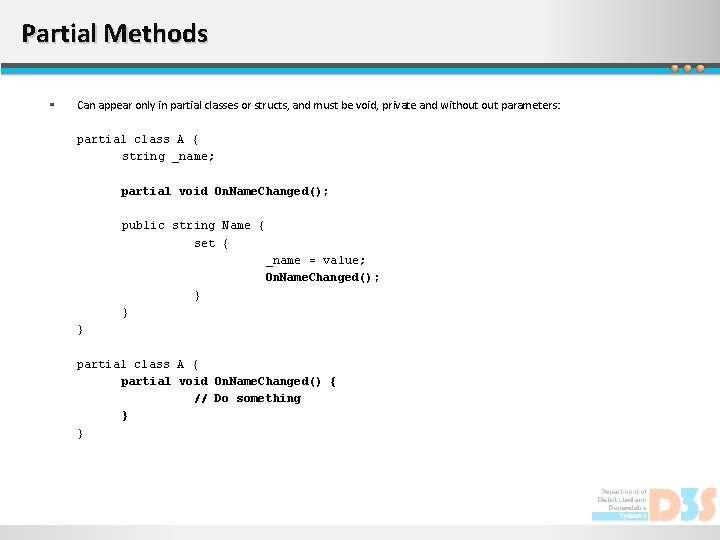Partial Methods Can appear only in partial classes or structs, and must be void, private and without parameters: partial class A { string _name; partial void On. Name. Changed(); public string Name { set { _name = value; On. Name. Changed(); } } } partial class A { partial void On. Name. Changed() { // Do something } }